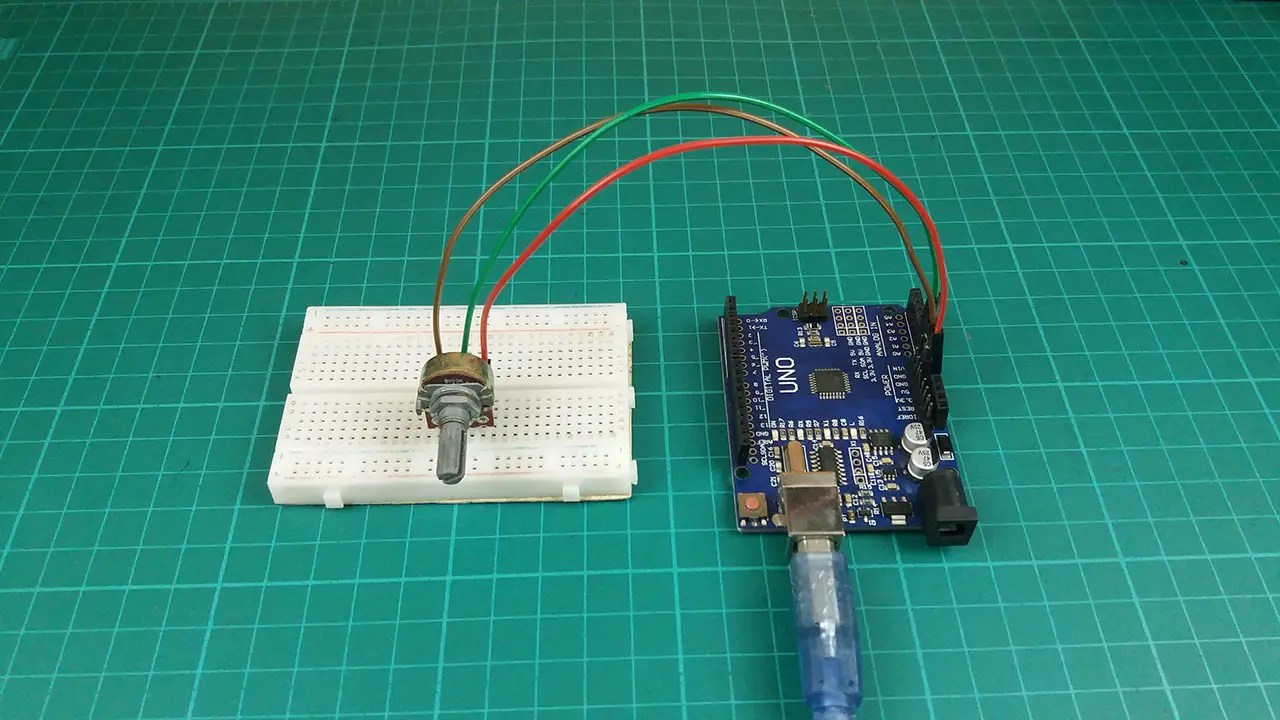Hello guys, Welcome back to my blog. I explained in the last article how to get analog outputs. In previous articles, I have explained how to get the output from the Arduino board. Today we are going to show you how to get input from the Arduino board. We can do it easily. I have used analog pins from the Arduino board for this project. The Arduino UNO and Arduino NANO boards have six analog pins. These pins are numbered from A0 to A5. One analog PIN gives a value from 0 to 1024. This is called an analog read. We can get an analog reading by connecting the sensors and external parts to the Arduino board. Okay, let’s do it practically. I have used a potentiometer (variable resistor) for this project and I’m used the serial monitor for watching reading. So let’s see doing this project step by step. The required components are given below.

### Components

Step 1

Arduino UNOI have used the Arduino Uno board for this project
Potentiometer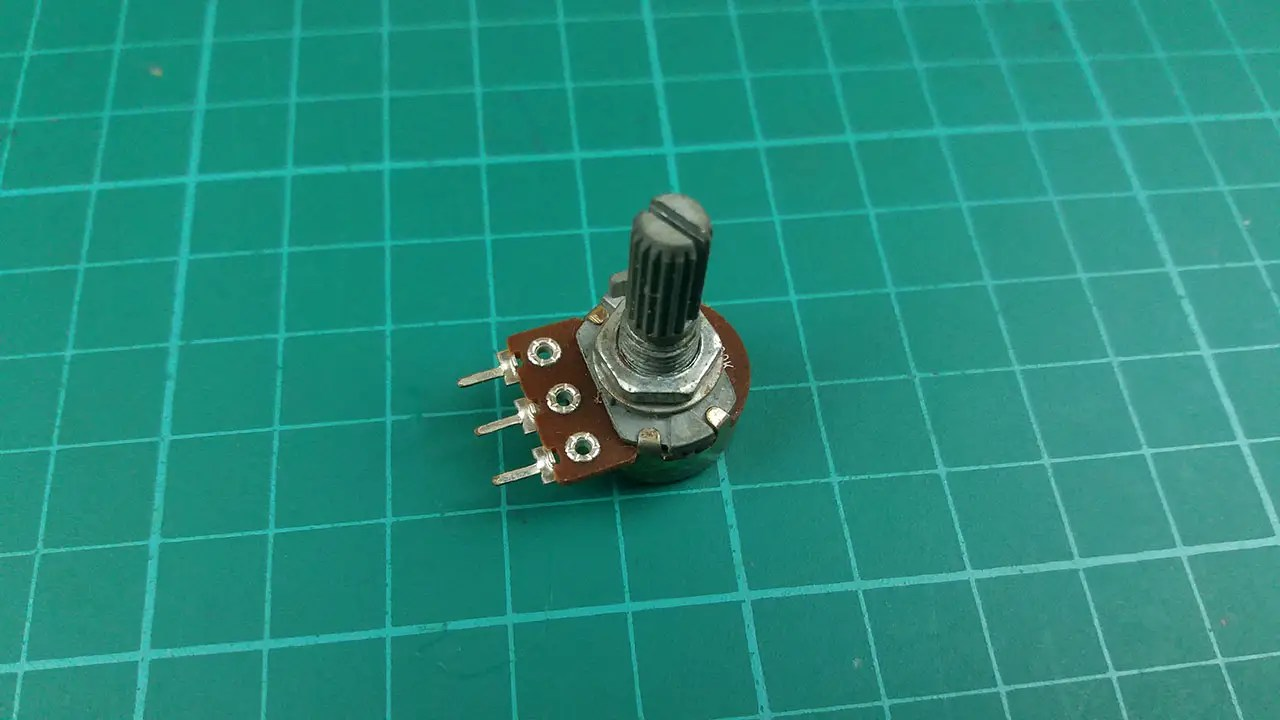It’s called a variable resistor.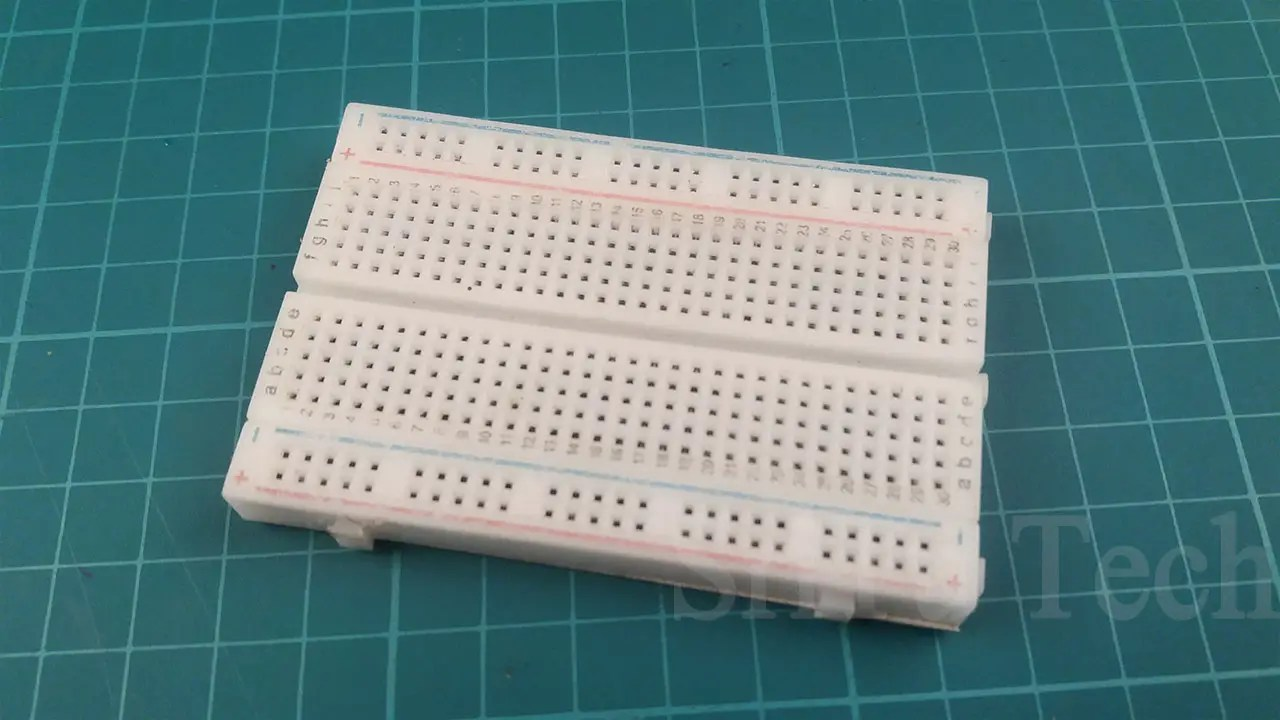It is most useful for testing circuits.
Jumper Wires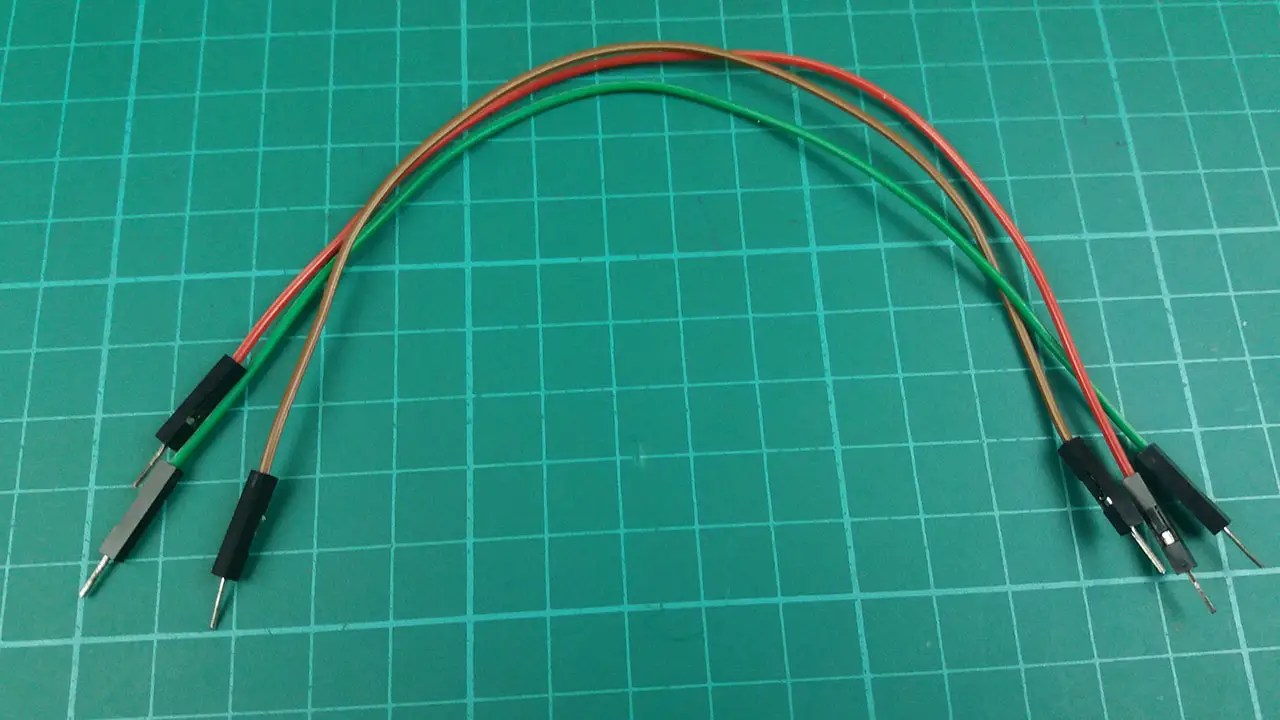I have used three male-to-male jumper wires.

Step 2
Connect the potentiometer to the breadboard.Step 3
Connect this circuit to the Arduino board. I have used A0 analog pin for this project. This pin should be attached to the center of the potentiometer. The other pins on the potentiometer must be attached to the VCC and GND pins.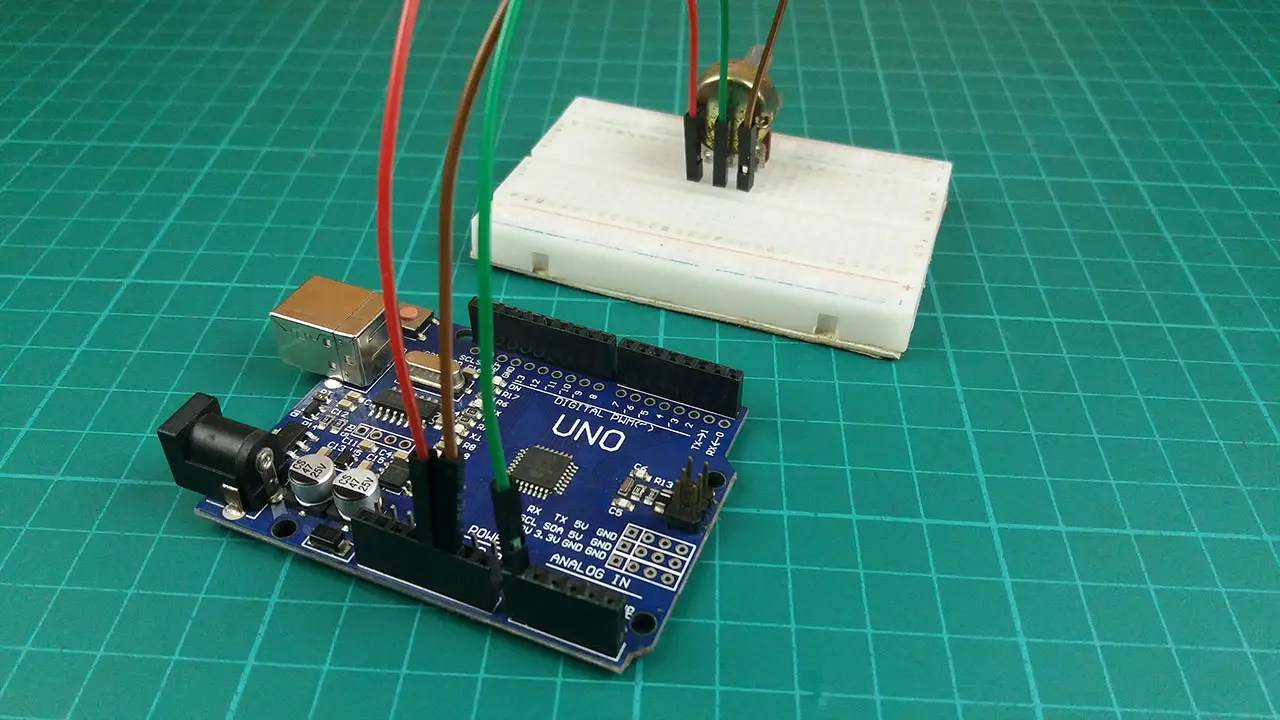Step 4

Below is the code I created.``````void setup() {
Serial.begin(9600);//enable serial monitor
}
void loop() {
int value = analogRead(A0);//Read the analog value and put it in the variable
Serial.println(value);//Print value
}``````
The serial monitor is enabled by the code of the void setup function. The void loop code reads the analog value and puts it into the variable.
int value – This code creates an integer variable. Its name is value. The value of the analog readings in this variable is stored.
analogRead(A0); – This code reads the analog value of the sensor or components connected to the A0 pin. This value is then added to the variable.
serial.println(value); – This code prints the value of the variable in the serial monitor.

Step 5
Then verify this code.Step 6
Select the Arduino board and port.Step 7
Finally, upload this code and run a serial monitor.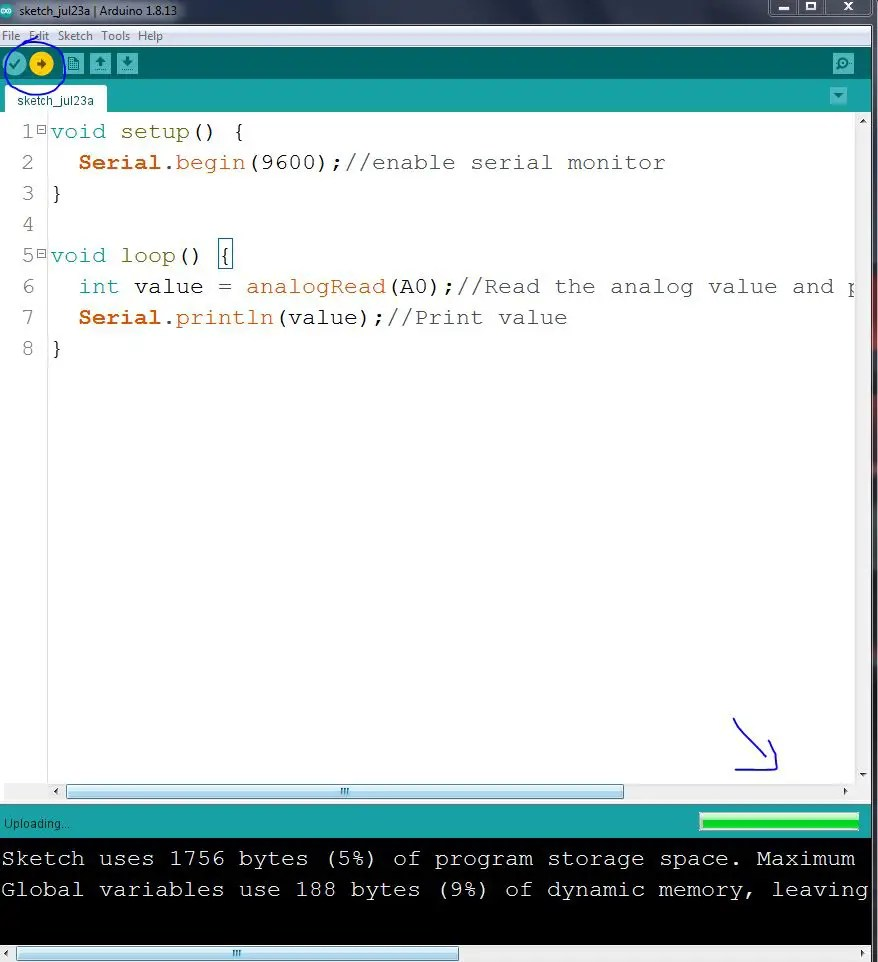OK did this project. Rotate the potentiometer and see how the values ​​change. It is displayed on the serial monitor.
Watch the video below.
Below is how the project will be carried out by the Arduino Nano board. Previous article knowledge has also been used for this project.OK let’s see how it is done step by step. For that, I used a potentiometer and an LED bulb. This project is done so that the LED brightness goes up and down when the potentiometer is rotated.
Step 1
Circuit diagram.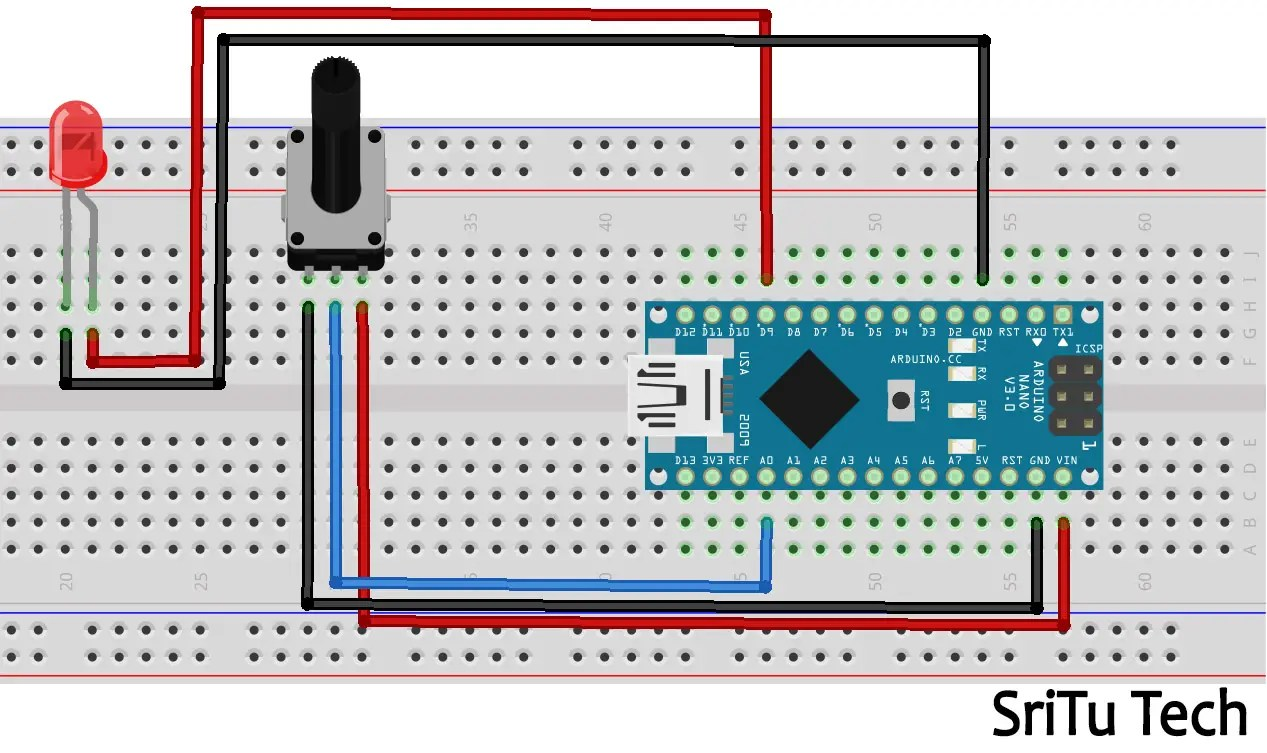Step 2
Source code
``````void setup() {
pinMode(9, OUTPUT);//define pin(PWM Pin)
Serial.begin(9600);//serial monitor enable
}
void loop() {
int brightness;//naming the veriable
brightness = map(brightness, 0, 1023, 0 , 255);//0-1023 to 0-255
analogWrite(9, brightness);//led on and off
Serial.println(brightness);//print serial monitor value
}``````
Step 3
Select board and port.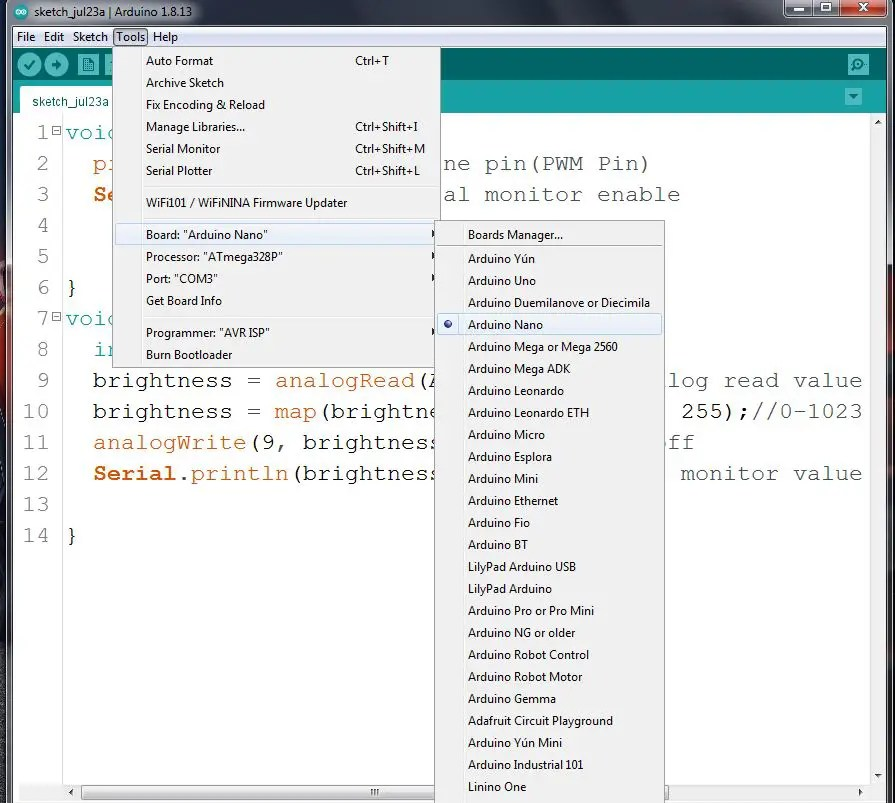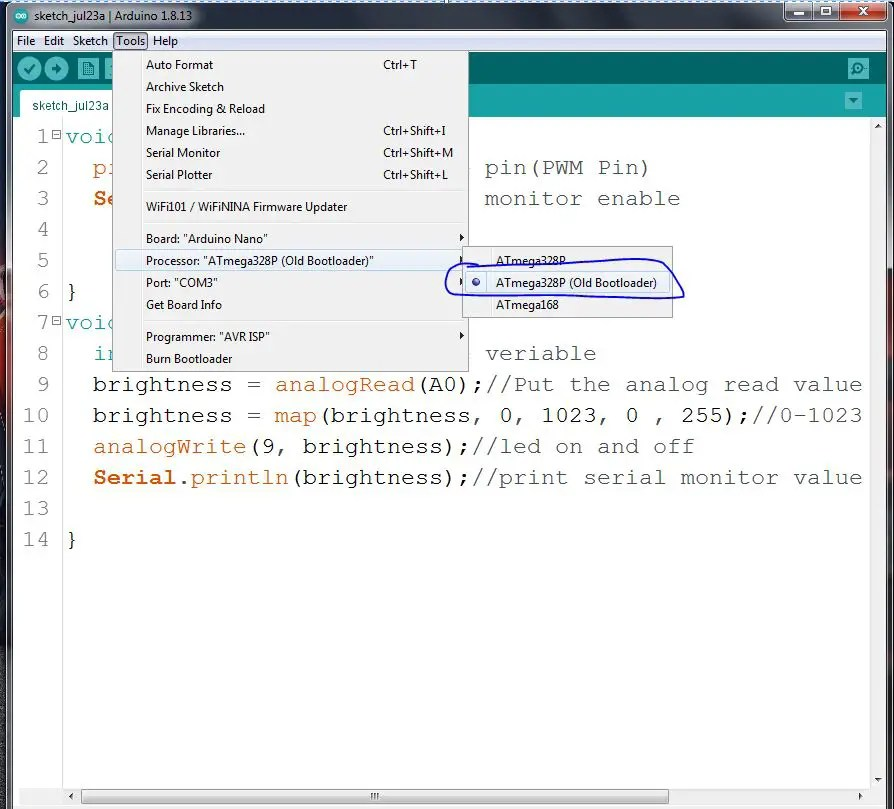Step 4